Скачать презентацию CSEP 590 Model Checking and Automated Verification

879729889feeee5fd86c0c1d08f84f13.ppt

• Количество слайдов: 12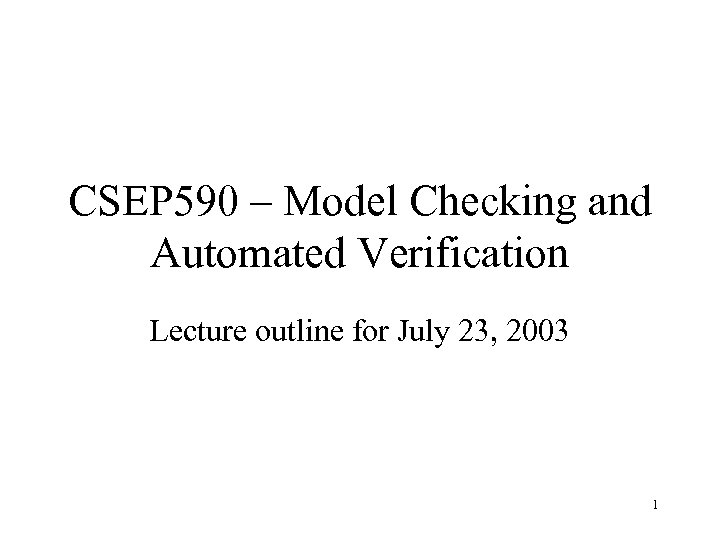CSEP 590 – Model Checking and Automated Verification Lecture outline for July 23, 2003 1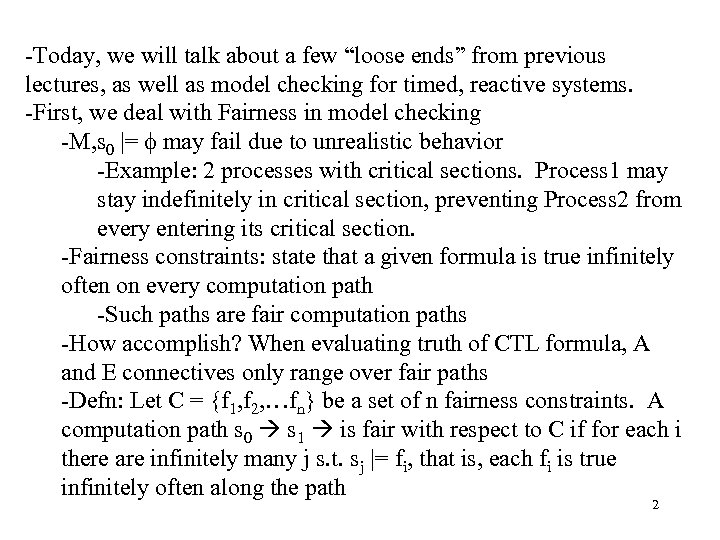-Today, we will talk about a few “loose ends” from previous lectures, as well as model checking for timed, reactive systems. -First, we deal with Fairness in model checking -M, s 0 |= may fail due to unrealistic behavior -Example: 2 processes with critical sections. Process 1 may stay indefinitely in critical section, preventing Process 2 from every entering its critical section. -Fairness constraints: state that a given formula is true infinitely often on every computation path -Such paths are fair computation paths -How accomplish? When evaluating truth of CTL formula, A and E connectives only range over fair paths -Defn: Let C = {f 1, f 2, …fn} be a set of n fairness constraints. A computation path s 0 s 1 is fair with respect to C if for each i there are infinitely many j s. t. sj |= fi, that is, each fi is true infinitely often along the path 2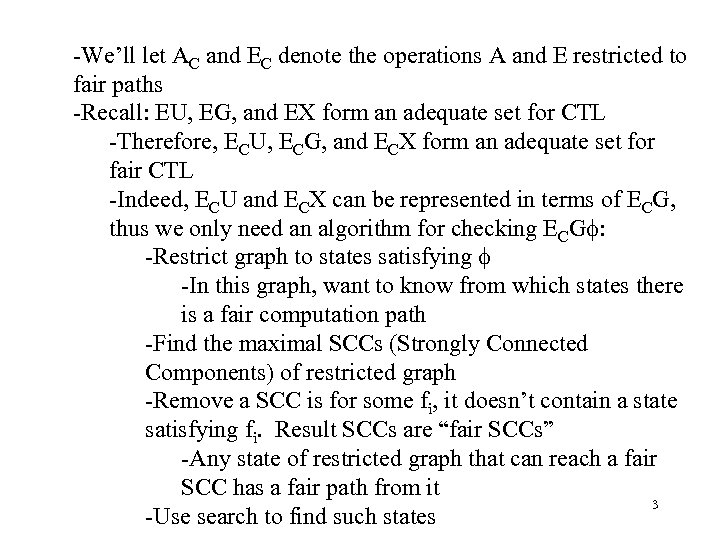-We’ll let AC and EC denote the operations A and E restricted to fair paths -Recall: EU, EG, and EX form an adequate set for CTL -Therefore, ECU, ECG, and ECX form an adequate set for fair CTL -Indeed, ECU and ECX can be represented in terms of ECG, thus we only need an algorithm for checking ECG : -Restrict graph to states satisfying -In this graph, want to know from which states there is a fair computation path -Find the maximal SCCs (Strongly Connected Components) of restricted graph -Remove a SCC is for some fi, it doesn’t contain a state satisfying fi. Result SCCs are “fair SCCs” -Any state of restricted graph that can reach a fair SCC has a fair path from it 3 -Use search to find such states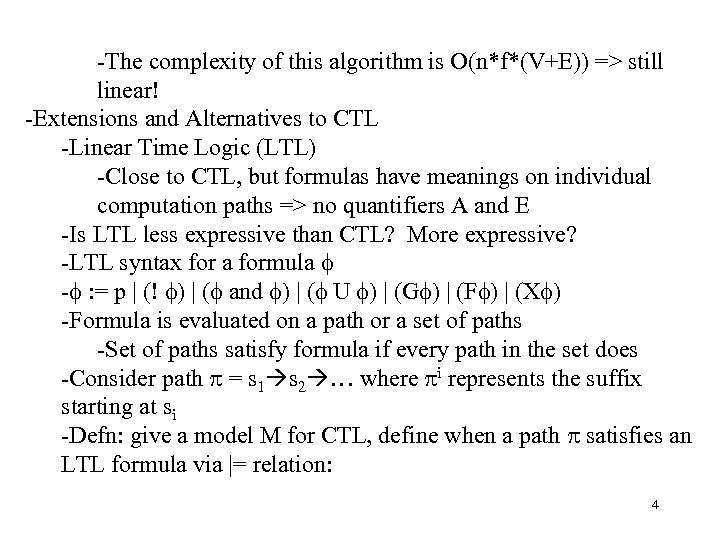-The complexity of this algorithm is O(n*f*(V+E)) => still linear! -Extensions and Alternatives to CTL -Linear Time Logic (LTL) -Close to CTL, but formulas have meanings on individual computation paths => no quantifiers A and E -Is LTL less expressive than CTL? More expressive? -LTL syntax for a formula - : = p | (! ) | ( and ) | ( U ) | (G ) | (F ) | (X ) -Formula is evaluated on a path or a set of paths -Set of paths satisfy formula if every path in the set does -Consider path = s 1 s 2 … where i represents the suffix starting at si -Defn: give a model M for CTL, define when a path satisfies an LTL formula via |= relation: 4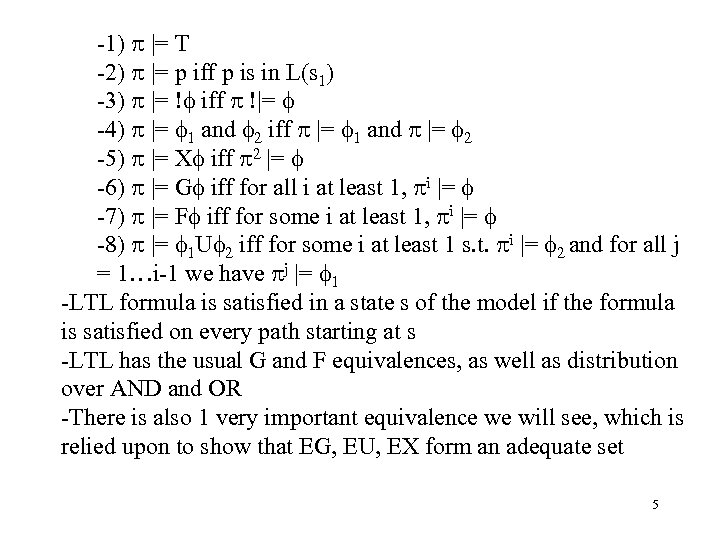-1) |= T -2) |= p iff p is in L(s 1) -3) |= ! iff !|= -4) |= 1 and 2 iff |= 1 and |= 2 -5) |= X iff 2 |= -6) |= G iff for all i at least 1, i |= -7) |= F iff for some i at least 1, i |= -8) |= 1 U 2 iff for some i at least 1 s. t. i |= 2 and for all j = 1…i-1 we have j |= 1 -LTL formula is satisfied in a state s of the model if the formula is satisfied on every path starting at s -LTL has the usual G and F equivalences, as well as distribution over AND and OR -There is also 1 very important equivalence we will see, which is relied upon to show that EG, EU, EX form an adequate set 5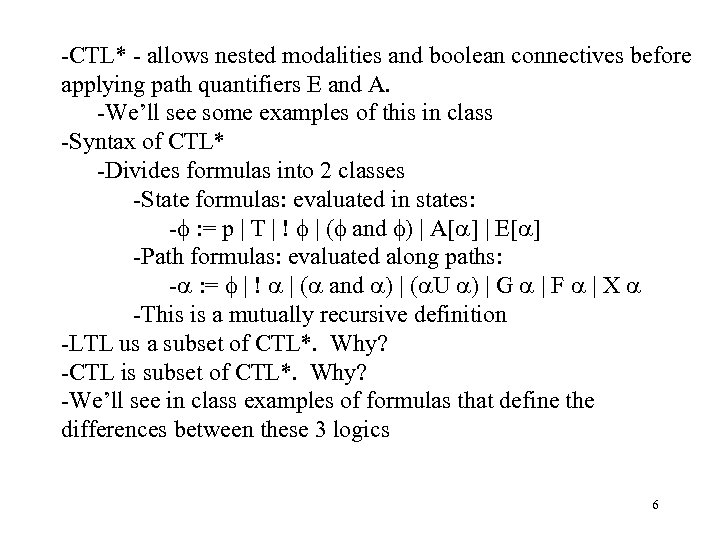-CTL* - allows nested modalities and boolean connectives before applying path quantifiers E and A. -We’ll see some examples of this in class -Syntax of CTL* -Divides formulas into 2 classes -State formulas: evaluated in states: - : = p | T | ! | ( and ) | A[ ] | E[ ] -Path formulas: evaluated along paths: - : = | ! | ( and ) | ( U ) | G | F | X -This is a mutually recursive definition -LTL us a subset of CTL*. Why? -CTL is subset of CTL*. Why? -We’ll see in class examples of formulas that define the differences between these 3 logics 6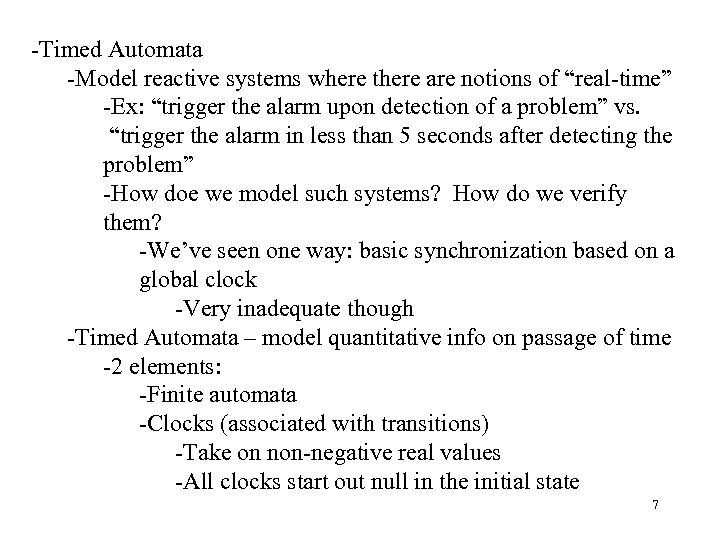-Timed Automata -Model reactive systems where there are notions of “real-time” -Ex: “trigger the alarm upon detection of a problem” vs. “trigger the alarm in less than 5 seconds after detecting the problem” -How doe we model such systems? How do we verify them? -We’ve seen one way: basic synchronization based on a global clock -Very inadequate though -Timed Automata – model quantitative info on passage of time -2 elements: -Finite automata -Clocks (associated with transitions) -Take on non-negative real values -All clocks start out null in the initial state 7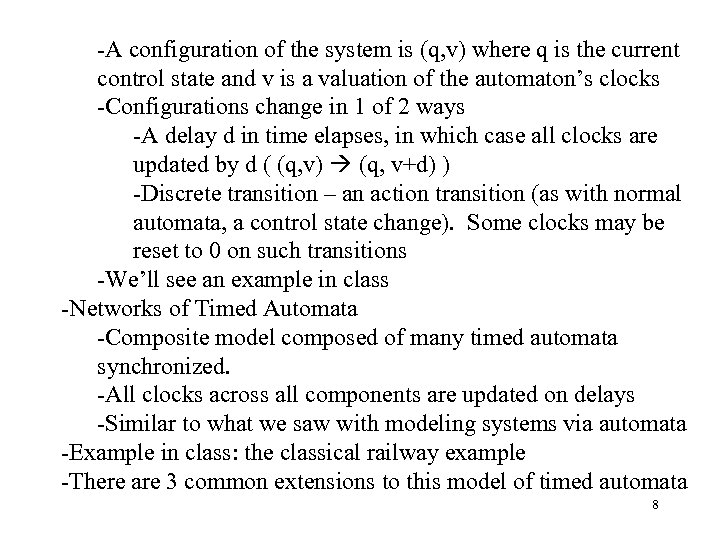-A configuration of the system is (q, v) where q is the current control state and v is a valuation of the automaton’s clocks -Configurations change in 1 of 2 ways -A delay d in time elapses, in which case all clocks are updated by d ( (q, v) (q, v+d) ) -Discrete transition – an action transition (as with normal automata, a control state change). Some clocks may be reset to 0 on such transitions -We’ll see an example in class -Networks of Timed Automata -Composite model composed of many timed automata synchronized. -All clocks across all components are updated on delays -Similar to what we saw with modeling systems via automata -Example in class: the classical railway example -There are 3 common extensions to this model of timed automata 8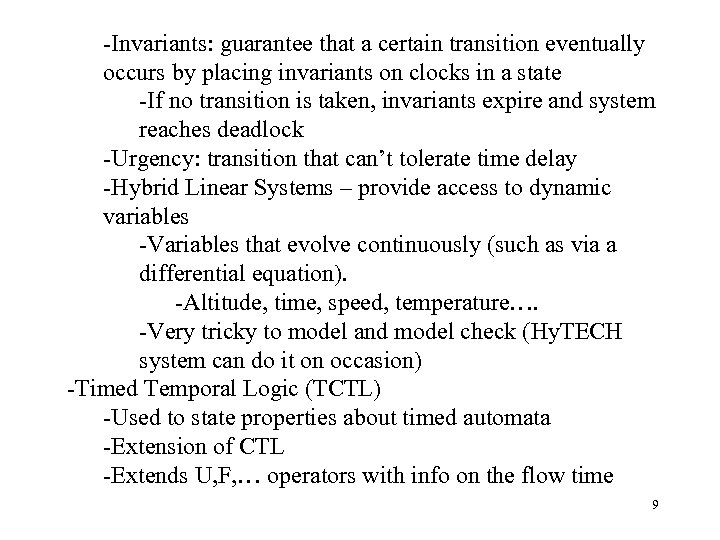-Invariants: guarantee that a certain transition eventually occurs by placing invariants on clocks in a state -If no transition is taken, invariants expire and system reaches deadlock -Urgency: transition that can’t tolerate time delay -Hybrid Linear Systems – provide access to dynamic variables -Variables that evolve continuously (such as via a differential equation). -Altitude, time, speed, temperature…. -Very tricky to model and model check (Hy. TECH system can do it on occasion) -Timed Temporal Logic (TCTL) -Used to state properties about timed automata -Extension of CTL -Extends U, F, … operators with info on the flow time 9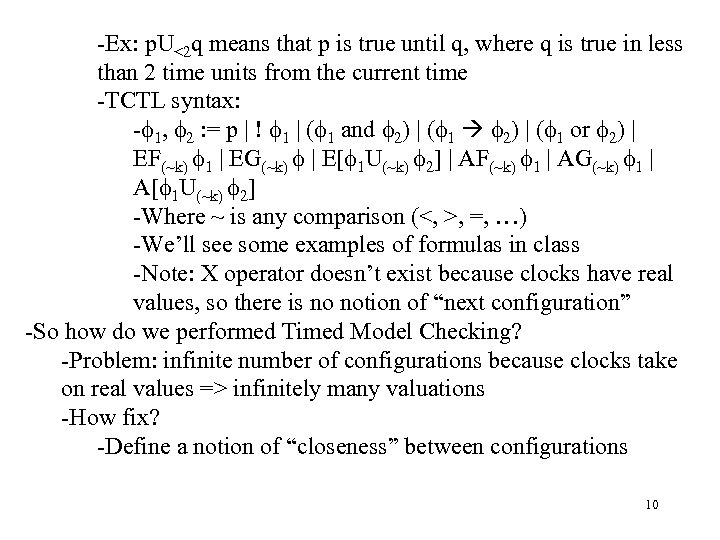-Ex: p. U<2 q means that p is true until q, where q is true in less than 2 time units from the current time -TCTL syntax: - 1, 2 : = p | ! 1 | ( 1 and 2) | ( 1 or 2) | EF(~k) 1 | EG(~k) | E[ 1 U(~k) 2] | AF(~k) 1 | AG(~k) 1 | A[ 1 U(~k) 2] -Where ~ is any comparison (<, >, =, …) -We’ll see some examples of formulas in class -Note: X operator doesn’t exist because clocks have real values, so there is no notion of “next configuration” -So how do we performed Timed Model Checking? -Problem: infinite number of configurations because clocks take on real values => infinitely many valuations -How fix? -Define a notion of “closeness” between configurations 10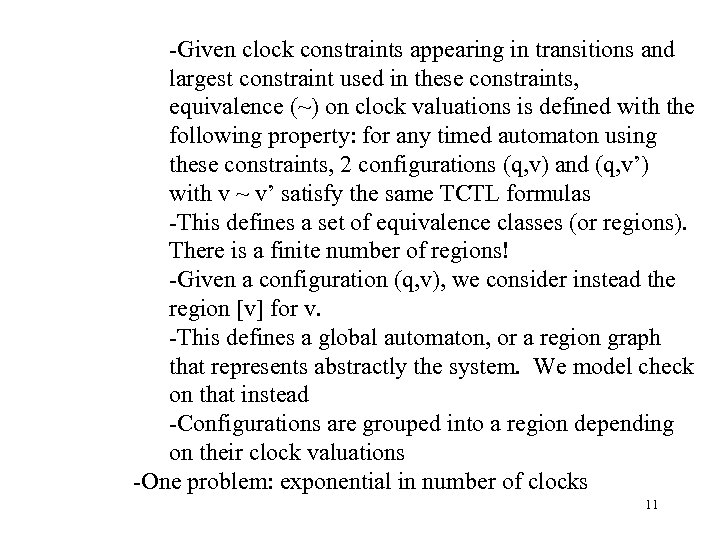-Given clock constraints appearing in transitions and largest constraint used in these constraints, equivalence (~) on clock valuations is defined with the following property: for any timed automaton using these constraints, 2 configurations (q, v) and (q, v’) with v ~ v’ satisfy the same TCTL formulas -This defines a set of equivalence classes (or regions). There is a finite number of regions! -Given a configuration (q, v), we consider instead the region [v] for v. -This defines a global automaton, or a region graph that represents abstractly the system. We model check on that instead -Configurations are grouped into a region depending on their clock valuations -One problem: exponential in number of clocks 11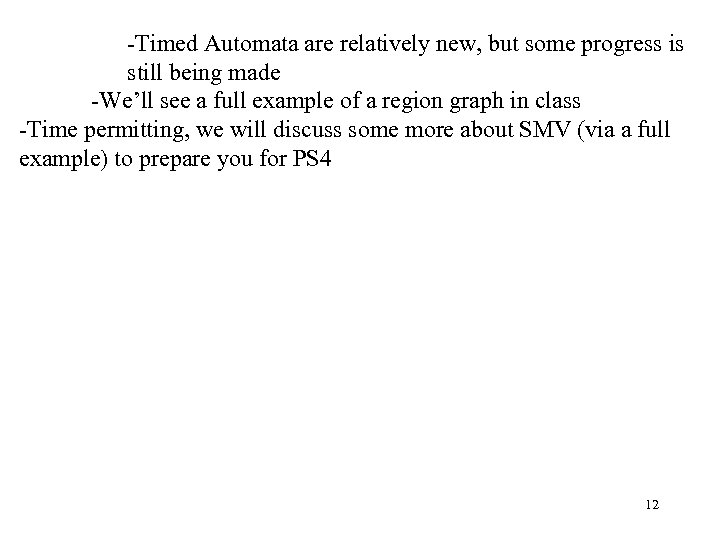-Timed Automata are relatively new, but some progress is still being made -We’ll see a full example of a region graph in class -Time permitting, we will discuss some more about SMV (via a full example) to prepare you for PS 4 12